# MCQs in Power Supplies (Voltage Regulators)

(Last Updated On: December 8, 2017)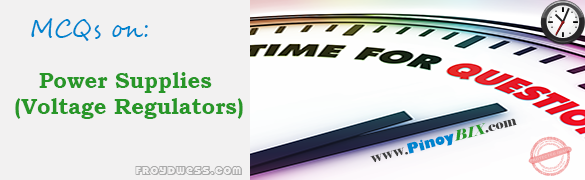This is the Multiple Choice Questions in Power Supplies (Voltage Regulators) from the book Electronic Devices and Circuit Theory 10th Edition by Robert L. Boylestad. If you are looking for a reviewer in Electronics Engineering this will definitely help. I can assure you that this will be a great help in reviewing the book in preparation for your Board Exam. Make sure to familiarize each and every questions to increase the chance of passing the ECE Board Exam.

### Online Questions and Answers Topic Outline

• MCQs in Introduction to Power Supplies | MCQs in General Filter Considerations | MCQs in Capacitor Filter | MCQs in RC Filter | MCQs in Discrete Transistor Voltage Regulation | MCQs in IC Voltage Regulators

### Practice Exam Test Questions

Choose the letter of the best answer in each questions.

1. In which of the following applications is a pulsating dc voltage suitable?

• A. Battery charger
• C. Stereo system
• D. Computer

2. Calculate the ripple of a filter output having a 20-V dc component and a 1.7 Vr(rms) ac component.

• A. 6%
• B. 8.5%
• C. 85%
• D. 58%

3. Calculate the voltage regulation of a power supply having VNL = 50 V and VFL = 48 V.

• A. 4.17%
• B. 5.2%
• C. 6.2%
• D. 7.1%

4. Across which of the following components of a power supply does the average (dc) voltage exist?

• A. Diodes
• B. Secondary of the transformer
• C. Capacitor filter
• D. None of the above

5. Calculate the ripple voltage of a full-wave rectifier with a 75-µF filter capacitor connected to a load drawing 40 mA.

• A. 1.20 V
• B. 1.28 V
• C. 1.32 V
• D. 1.41 V

6. What is the ratio of the period of the output voltage to the period of the input voltage in a full-wave rectifier?

• A. 0
• B. 0.5
• C. 1
• D. 2

7. A _____ -wave rectified signal has less ripple than a _____ -wave rectified signal and is thus better to apply to a filter.

• A. full, half
• B. half, full

8. If the value of full-load voltage is the same as the no-load voltage, the voltage regulation calculated is _____ %, which is the best expected.

• A. 0
• B. 1
• C. 99
• D. 100

9. In which period is the capacitor filter charged in a full-wave rectifier?

• A. The time during the positive cycle
• B. The time during which the diodes are not conducting
• C. The time during which the diode(s) is (are) conducting
• D. The time during the negative cycle

10. In which period is the capacitor filter discharged through the load in a full-wave rectifier?

• A. The time during the positive cycle
• B. The time during which the diodes are not conducting
• C. The time during which the diode(s) is (are) conducting
• D. The time during the negative cycle

11. If a peak rectified voltage for the full-wave filter circuit is 40 V, calculate the filter dc voltage if C = 75 µF and load current is 40 mA.

• A. 27.9 V
• B. 32.12 V
• C. 37.78 V
• D. 40 V

12. Calculate the ripple of a capacitor filter for a peak rectified voltage of 40 V, a capacitor value C = 75 µF, and a load current of 40 mA.

• A. 3.2%
• B. 3.59%
• C. 4.03%
• D. 4.59%

13. What is the ratio of the peak ripple voltage level to its rms voltage level?

• A. √3
• B. √2
• C. √3/2
• D. √2/2

14. The larger the value of the capacitor, the smaller the peak current drawn through the rectifying diodes.

• A. True
• B. False

15. What is the purpose of an additional RC filter section in a power supply circuit?

• A. Increase the dc voltage component
• B. Increase the ac voltage component
• C. Decrease the ac voltage component
• D. None of the above

16. Calculate the dc voltage across a 2-kΩ load for an RC filter section (R = 50 Ω, C = 20 µF). The dc voltage across the initial filter capacitor is Vdc = 50 V.

• A. 40.78 V
• B. 42.78 V
• C. 45.78 V
• D. 48.78 V

17. The purpose of the added RC section is to pass most of the dc component while reducing as much of the ac component as possible.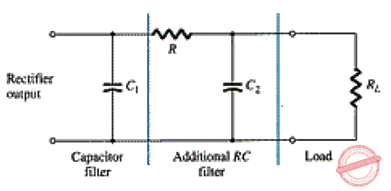• A. True
• B. False

18. This circuit is an example of the ac equivalent of an RC filter.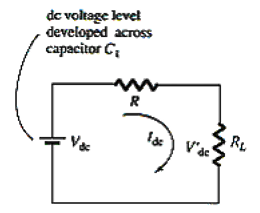• A. True
• B. False

19. For a full-wave rectifier with ac ripple at 120 Hz, the impedance of a capacitor can be calculated using XC = _____.

• A. 0.707 ÷ C
• B. 1.414 ÷ C
• C. 1.3 ÷ C
• D. 0.785 ÷ C

20. In a simple series regulator circuit, which of the following components is the controlling element?

• A. Load resistor
• B. Zener diode
• C. Transistor Q1
• D. None of the above

21. In this improved series regulator circuit, which of the following components is the sampling circuit?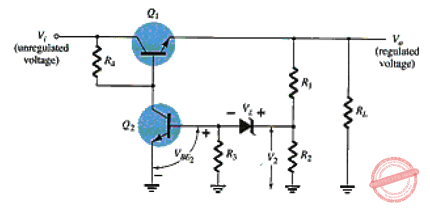• A. Zener diode
• B. Load resistor
• C. Either of the two transistors Q1 or Q2
• D. Resistors R1 and R2

22. In this op-amp series regulator circuit, which of the following components is the comparator circuit?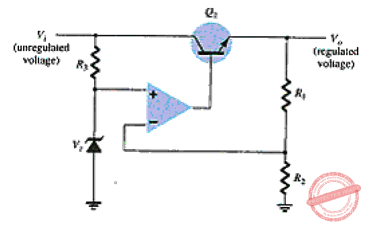• A. Op-amp
• B. Transistor Q1
• C. R1 and R2 resistors
• D. Zener diode

23. What regulated output voltage is provided for the following circuit elements: R1 = 15 kΩ, R2 = 35 kΩ, and VZ = 11.2 V?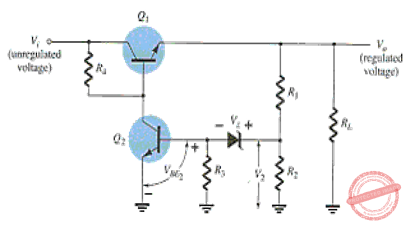• A. 16.50 V
• B. 17 V
• C. 17.35 V
• D. 18.25 V

24. Which component(s) set(s) the voltage across the load in a basic transistor shunt regulator?

• A. Zener diode
• B. Transistor base-emitter voltage
• C. Both the Zener diode and the transistor base-emitter voltage
• D. None of the above

25. In an improved shunt regulator, which of the following components sets the reference voltage?

• A. Transistor Q1
• B. Zener diode
• C. Transistor Q2
• D. RS

26. For what range of load current can voltage regulators be selected for operation?

• A. Hundreds of picoamperes to tens of nanoamperes
• B. Hundreds of picoamperes to tens of milliamperes
• C. Hundreds of milliamperes to tens of amperes
• D. None of the above

27. For what range of fixed regulated voltages do the series 78xx regulators provide regulation?

• A. –5 V to +24 V
• B. +5 V to +24 V
• C. –5 V to –24 V
• D. None of the above

28. What is the typical dropout voltage for the 7812 fixed positive voltage regulator?

• A. 4 mV
• B. 100 mV
• C. 1.5 V
• D. 2 V

29. How many diodes conduct in the full-wave bridge rectifier while the capacitor is being charged?

• A. 1
• B. 2
• C. 3
• D. 4

30. What is the range of the voltage level of the LM317 adjusted voltage regulator?

• A. 0 V to 5 V
• B. 1.2 V to 37 V
• C. –5 V to –24 V
• D. 5 V to 24 V

31. What are the typical values of Vref and Iadj for the LM317 adjustable voltage regulator?

• A. 1.0 V, 100 mA
• B. 1.5 V, 100 mA
• C. 1.25 V, 100 μA
• D. 1.25 V, 10 mA

32. The 7812 regulator IC provides _____.

• A. 5 V
• B. –5 V
• C. 12 V
• D. –12 V

33. The 7912 regulator IC provides _____ .

• A. 5 V
• B. –5 V
• C. 12 V
• D. –12 V

34. The 7905 regulator IC provides _____.

• A. 5 V
• B. –5 V
• C. 12 V
• D. –12 V

35. The 7805 regulator IC provides _____.

• A. 5 V
• B. –5 V
• C. 12 V
• D. –12 V

#### FILL-IN-THE-BLANKS

1. A complete power supply has a _____.

• A. rectifier
• B. filter
• C. voltage regulator
• D. All of the above

2. The output resulting from a rectifier is a(n) _____.

• A. ac voltage
• B. pure dc voltage
• C. pulsating dc voltage
• D. None of the above

3. The _____ the ac variation with respect to the dc level, the _____ the filter circuit’s operation.

• A. smaller, better
• B. larger, better
• C. smaller, worse
• D. None of the above

4. A dc voltmeter reads the _____ while measuring a pulsating dc voltage.

• A. ac component of the signal
• B. average of the signal
• C. peak of the pulsating signal
• D. None of the above

5. The filter output voltage of a power supply _____ when load current is drawn from the supply.

• A. remains the same
• B. is increased
• C. is reduced
• D. None of the above

6. The _____ the voltage regulation, the _____ the operation of the voltage supply circuit.

• A. smaller, better
• B. larger, better
• C. smaller, worse
• D. None of the above

7. A full-wave rectified signal has _____ dc component and _____ ripple than (as) the half-wave rectified voltage.

• A. a larger, more
• B. a smaller, less
• C. the same, less
• D. a larger, less

8. If the value of the full-load voltage is the same as the no-load voltage, the voltage regulation calculated is _____.

• A. 0%
• B. a negative percentage
• C. a positive percentage
• D. None of the above

9. In a half-wave rectifier, the dc voltage level is _____ the ripple voltage level.

• A. smaller than
• B. the same as
• C. larger than
• D. None of the above

10. In a full-wave rectifier, the dc voltage level is _____ the ripple voltage level.

• A. smaller than
• B. the same as
• C. larger than
• D. None of the above

11. In a full-wave rectifier, if no load were connected across the capacitor, the output voltage would ideally be a(n) _____.

• A. ac voltage
• B. constant dc voltage
• C. pulsating dc voltage
• D. ramp voltage

12. The output of a loaded power supply is _____ that of the unloaded.

• A. the same as
• B. larger than
• C. smaller than
• D. None of the above

13. The charging and discharging of the capacitor filter take _____ of the period of the input voltage.

• A. 0.25
• B. 0.5
• C. 0.75
• D. 1

14. The frequency of the output voltage of a full-wave rectifier is _____ the frequency of its input voltage.

• A. the same as
• B. twice
• C. one-half
• D. one-third

15. The ripple voltage Vr is a result of the _____.

• A. conduction of the diode(s)
• B. transformer windings
• C. charging and discharging of the capacitor
• D. load resistor

16. The _____ values of capacitor filter provide _____ ripple and _____ average voltage.

• A. larger, more, higher
• B. smaller, less, lower
• C. smaller, more, higher
• D. larger, less, higher

17. The _____ the diode conduction time, the _____ the amount of the charging current through the capacitor filter.

• A. shorter, larger
• B. shorter, smaller
• C. longer, larger
• D. None of the above

18. In a current-limiting circuit, _____ provide(s) the limiting of the maximum load current.

• A. the Zener diode
• B. the short-circuit resistor Rsc
• C. the transistor Q1
• D. both the short-circuit resistor Rsc and transistor Q2

19. In a foldback configuration, limiting the current reduces _____, protecting the load from overcurrent as well as protecting the regulator.

• A. the output voltage
• B. the output current
• C. both the output voltage and output current
• D. None of the above

20. A type of regulator circuit that is quite popular for its efficient transfer of power to the load is the _____.

• A. current-limiting voltage regulator
• B. switching regulator
• C. foldback limiting regulator
• D. op-amp series regulator

21. Regulator IC units contain the circuitry for the _____.

• A. reference source
• B. comparator amplifier
• C. control device and overload protection
• D. All of the above

22. IC units provide regulation of _____.

• A. a fixed positive voltage
• B. a fixed negative voltage
• C. an adjustably set voltage
• D. All of the above

23. The specification sheet for the 7812 fixed positive voltage regulator shows that the output voltage could be as low as _____ or as high as _____.

• A. 11.5 V, 12.5 V
• B. 11.2 V, 12.2 V
• C. 11 V, 13 V
• D. 8 V, 15 V

24. The series 7900 ICs are _____.

• A. positive voltage regulators
• B. negative voltage regulators
• C. both positive and negative voltage regulators
• D. adjustable-set voltage regulators

25. The _____ is (are) an adjustable voltage regulator.

• A. series 7800 ICs
• B. series 7900 ICs
• C. LM317
• D. None of the above

Rate this: